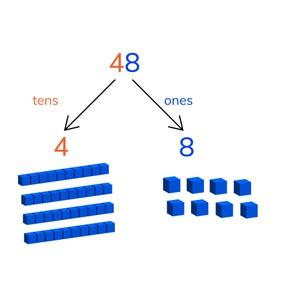Place value- numbers to 100

# Place value- numbers to 100

Place value- numbers to 100

No account needed.8,000 schools use Gynzy92,000 teachers use Gynzy1,600,000 students use Gynzy

## General

Students learn place value of numbers from 20to 99. They learn that the numbers 20-99 are made of tens and ones.

-1.NBT.B.2

## Relevance

Discuss with students that it is important to know place value in numbers to 100, so you can learn to calculate with these numbers.

## Introduction

The interactive whiteboard has a few numbers. Point at a number and ask a student to say out loud which number it is. Repeat this with all numbers. Next the students must count the amount on the interactive whiteboard. Ask the students if they know any tricks to count faster.

## Development

Using MAB blocks explain what tens and ones are. Explain that tens are a group of 10 and ones are the numbers from 1 to 9. Show MAB-blocks on the interactive whiteboard and/or show them in your classroom. Using the blocks clarify the explanation of tens and ones. Ask students to practice with MAB-blocks by setting the correct amount in front of them. You can also use the MAB-blocks on the interactive whiteboard. Next using the stamps explain what tens are, how many ones you have, and what the number together is. As a class fill in the missing numbers and practice naming tens and ones in numbers without visual support.

Check that students understand how to use place value to build numbers to 100 by asking the following questions:
What are tens? How do you know?
What are ones? How do you know?
Which tens and ones are in 68?

## Guided Practice

Students will first practice naming the tens and ones that make a number with visual support. Then they combine tens and ones to say which number is shown.

## Closing

Discuss with students that it is important to know how numbers to 100 work, so you can calculate with them later. Check that students understand tens and ones and that you can combine them. Ask students to write down which number needs to be filled in. The images given are to support their thinking. Ask students to hold up their answers so you can check their work.

## Teaching Tip

Students who have difficulty with place value can be supported with manipulatives like MAB blocks to help visualize the tens and ones.

## Instruction materials

MAB blocks

### The online teaching platform for interactive whiteboards and displays in schools

• Save time building lessons

• Manage the classroom more efficiently

• Increase student engagement